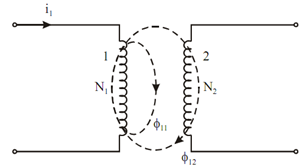## Inductance Assignment Help

Assignment Help: >> Properties of Conductors and Insulators - Inductance

Inductance:

Concept of inductance was introduced by Faraday in the year of 1831. Mutual inductance was the significant result of Faraday's well known law of electromagnetic induction. Before stating the mutual inductance, we will develop the concept of self induction which has already been discussed.

According to Faraday's law of electromagnetic inductance, when the magnetic flux connect with the coil (because of current in the same coil) changes with time then emf is induced in that coil. The magnitude of this induced emf may be expressed by VL or e) is specified by

νL = N  (dt/ d φ)                    -------- (1)

Where N = number of turns in the coil, and

φ = flux per turn (in weber)

From Eq. (1)

ν L = d ( N φ)/ dt = d ψ  / dt       ------ (2)

where Ψ = total flux connect with the coil (in weber)

However total flux ψ is directly proportional to the current i in the coil

∴ ψ∝ i

or, ψ= Li                   ---------- (3)

where L refer to the proportionality constant and known as the self inductance of coil. Unit of inductance is Henry.

Put the value of ψ from Eq. (3) to Eq. (2)

ν L         = L (di  / dt  )         ---------- (4)

In a similar manner, we may define the mutual inductance. Consider two isolated coils illustrated in Figure, in which coil 1 is carrying the current i1.Figure: Two Isolated Coils with Current i1

 Energy Stored in an Inductor Inductances in Series and Parallel Inductances in Terms of Reluctance Two Isolated Coils Types of Inductor